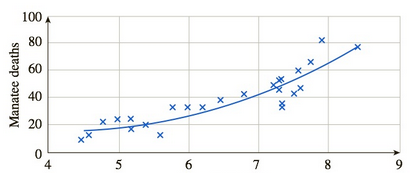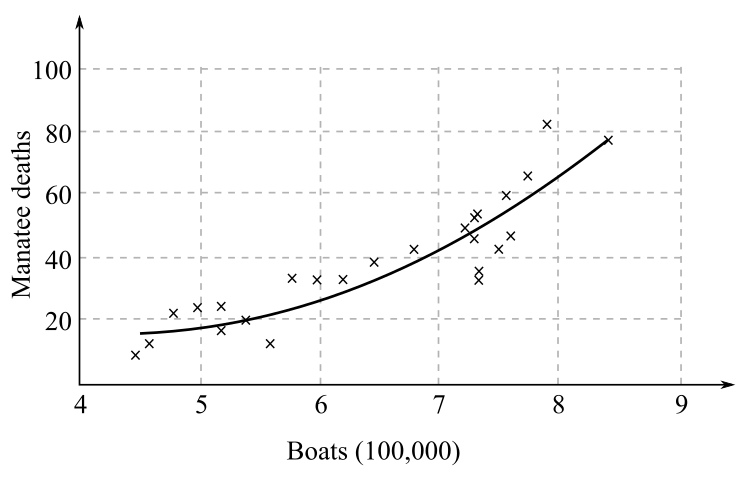Chapter 11.1, Problem 99EFinite Mathematics and Applied Cal...

7th Edition
Stefan Waner + 1 other
ISBN: 9781337274203

Solutions

Chapter
SectionFinite Mathematics and Applied Cal...

7th Edition
Stefan Waner + 1 other
ISBN: 9781337274203
Textbook Problem

Ecology Increasing numbers of manatees (“sea sirens”) have been killed by boats off the Florida coast. The following graph shows the relationship between the number of boats registered in Florida and the number of manatees killed each yearBoats (100,000).The regression curve shown in given by f ( x ) = 3.55 x 2 − 30.2 x + 81      ( 4.5 ≤ x ≤ 8.5 ) , where x is the number of boats (hundreds of thousands) registered in Florida in a particular year and f ( x ) is the number of manatees killed by boats in Florida that year.a. Find f ' ( x ) , and use your formula to compute f ' ( 8 ) , stating its units of measurement. What does the answer say about manatee deaths?b. Is f ' ( x ) increasingor decreasing with increasing x? Interpret the answer. [HINT: See Example 4.]

(a)

To determine

To calculate: The derivative f'(x) and f'(8) stating the units, where regression curve is given by f(x)=3.55x230.2x+81 where, f(x) is the number of manatees killed and x is the number of boats in hundreds of thousands which are registered in Florida dollars lies in the range 4.56x8.5. Also state the interpretation about the manatee death.Explanation

Given Information:

Regression curve is given by f(x)=3.55x230.2x+81 where, f(x) is the number of manatees killed and x is the number of boats in hundreds of thousands which are registered in Florida dollars lies in the range 4.56x8.5.

Formula used:

Sum and difference rule of derivative is [f±g](x)=f(x)±g(x).

Constant multiple rule is f(cx)=cf(x), where c is any constant.

Derivative of a constant is 0.

Power rule for the function f(x)=xn is f'(x)=nxn1 where n is any constant.

Calculation:

Consider the function, f(x)=3.55x230.2x+81

Apply sum and difference rule of derivative to the function f(x)=3.55x230.2x+81 with respect to x.

f(x)=ddx(3.55x2)ddx(30.2x)+ddx(81)

Apply constant multiple rule,

f(x)=3

(b)

To determine

Whether the derivative f'(x) is increasing or decreasing with increasing value of x where regression curve is given by f(x)=3.55x230.2x+81 where, f(x) is the number of manatees killed and x is the number of boats in hundreds of thousands which are registered in Florida dollars lies in the range 4.56x8.5.Still sussing out bartleby?

Check out a sample textbook solution.

See a sample solution

The Solution to Your Study Problems

Bartleby provides explanations to thousands of textbook problems written by our experts, many with advanced degrees!

Get Started

Evaluate the integral. 23(x23)dx

Calculus (MindTap Course List)

Name three types of reasoning.

Elementary Geometry For College Students, 7e

Simplify each expression. 342

Trigonometry (MindTap Course List)

For y=12gt2 (where g is a constant), dydt=. a) 14g2 b) 12g c) gt d) gt2

Study Guide for Stewart's Single Variable Calculus: Early Transcendentals, 8th